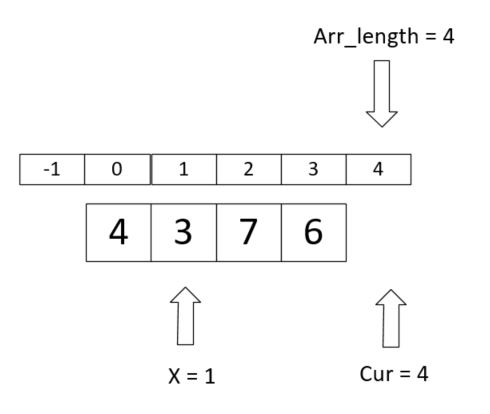# 左神算法基础class2——例子1荷兰国旗问题

• 时间:

### 左神算法基础class2——例子1荷兰国旗问题

• 预备题目：给定一个数组arr，和一个数num，请把小于等于num的数放在数组的左边，大于num的数放在数组的右边。要求额外空间复杂度O(1)，时间复杂度O(N)
• 分析
• 核心代码
• 完整代码
• 荷兰国旗问题：给定一个数组arr，和一个数num，请把小于num的数放在数组的左边，等于num的数放在数组的中间，大于num的数放在数组的右边。要求额外空间复杂度O(1)，时间复杂度O(N)
• 分析
• 完整代码

# 预备题目：给定一个数组arr，和一个数num，请把小于等于num的数放在数组的左边，大于num的数放在数组的右边。要求额外空间复杂度O(1)，时间复杂度O(N)

## 分析

1.把数组划分为小于等于num的区域和大于num的区域。用变量x代表小于区域的位置。刚开始由于不存在小于等于的区域，则`x = -1`,表示指向数组-1(不存在)。再设置变量cur作为遍历的位置，刚开始`cur = 0`指向数组第一个数。
2.开始遍历
（1）如果cur<=num ，那么把cur当前的元素和小于等于区域的下一个数交换，即++x的位置和cur位置的数交换，cur，x后移；
（2）如果cur>num，cur后移；
（3）如果cur == arr_length结束遍历。

eg [4,6,7,3],num = 5
1.cur 指向4，4<5,把4和x的下一位即0号位置4（自己和自己）交换。之后x指向4，cur++，cur指向6；
2.cur指向6，5<6,x不变，cur++；
3.cur指向7，5<7,x不变，cur++；
4.cur指向3，3<5,交换，x的下一位即6和3交换。之后x指向3，cur++，退出循环。## 核心代码

``````while(cur < arr_length)
{
if(arr[cur] <= num)
{
swap(arr[cur++],arr[++x]);
}
else
{
cur++;
}
}
``````

## 完整代码

``````#include<iostream>
#include<time.h>
#define arr_length 10
using namespace std;

//交换函数
void swap(int &a,int &b)
{
int temp = a;
a = b;
b = temp;
}

int main()
{
int arr[arr_length];
int cur = 0;
int num = 6;
int x = -1;

//生成随机长度为arr_length的数组，并输出
srand((unsigned)time(NULL));
cout<<"arr = ";
for(int i = 0;i < arr_length;i++)
{
arr[i] = rand()%10;
cout<<arr[i]<<" ";
}
cout<<endl;

//核心代码
while(cur < arr_length)
{
if(arr[cur] <= num)
{
swap(arr[cur++],arr[++x]);
}
else
{
cur++;
}
}

//输出交换后的arr1，arr2
cout<<"arr1 = ";
for(int i = 0;i<=x;i++)
{
cout<<arr[i]<<" ";
}
cout<<endl;
cout<<"arr2 = ";
for(int i = x+1;i<arr_length;i++)
{
cout<<arr[i]<<" ";
}
cout<<endl;
system("pause");
return 0;
}
``````